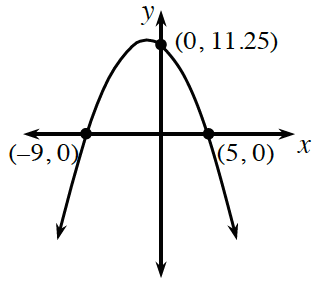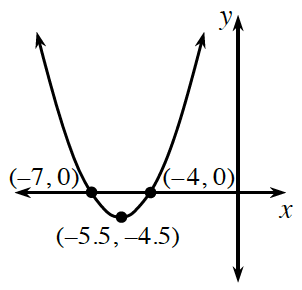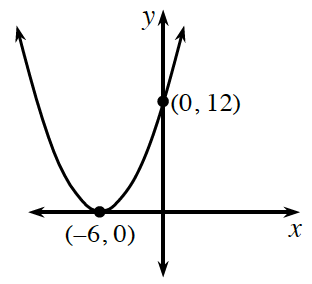### Home > INT2 > Chapter 9 > Lesson 9.3.4 > Problem9-122

9-122.

Based on the graph, write an equation for each parabola. 9-122 HW eTool

1.1.1.A quadratic equation forms a parabola when graphed. When $x$ equals $-9$ or $5$, $y$ equals $0$. This means that $-9$ and $5$ are roots of the equation. If you know the roots, can you find the factors for a possible equation?

The factors for a possible equation are
$(x + 9)$ and $(x - 5)$.

$y = (x + 9) (x - 5)$

See the hint for part (a).

See the hint for part (a). Because the parabola intersects the $x$-axis at only one point, its equation has a single factor, which is squared.

Use the eTool below to help you write and check a possible equation for each parabola.
Click on the link at right for the full eTool version: INT2 9-122 HW eTool